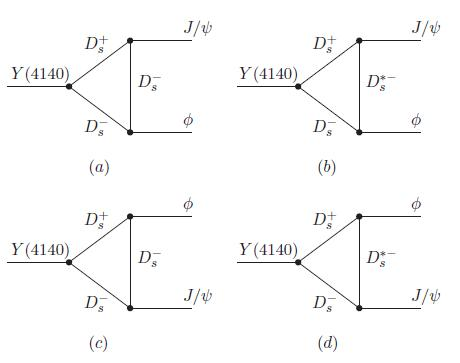## What is the Y(4140)? The plot thickensApril 6, 2009

Posted by dorigo in news, physics, science.
Tags: , , ,

I read with interest -but it would probably be more honest to say I browsed, since I could understand less than 50%- a preprint released three days ago on “The hidden charm decay of Y(4140) by the rescattering mechanism“, by Xiang Liu, from Peking University (now at Coimbra, PT). The Y particle has been recently discovered by CDF.

The existence of the several new resonances of masses above 3 GeV recently unearthed by B factories and by the CDF experiment poses a challenge to our interpretation of these states as simple quark-antiquark bound states, because of their properties -in particular, their decay pattern and their natural widths.

Already with the first “exotic” meson discovered a few years ago (and recently measured with great precision by CDF), the X(3872), the puzzle was evident: at a mass almost coincident with twice the mass of conventional charmed mesons (states which are labeled “D”, which are composed of two quarks: a charm and a up or down quark, like$D^+ = c \bar d$ or$D^\circ = c \bar u$), the X was immediately suggested to be a molecular state of two D particles. I wrote an account of the studies of the nature of the X particle a few years ago if you are interested -but mind you, the advancements in this research field are quick, and I believe the material I wrote back then is a bit aged by now.

The paper by Liu tries to determine whether the interpretation of the Y particle as a pure second radial excitation of P-wave charmonium ($\chi_{cJ}''$, with$J=0,1$) holds water once the observed branching ratio of the Y into the final state seen by CDF ($Y \to J/\psi \phi$), and the measured decay width, are compared to a theoretical calculation.

The nice thing about the decay of the Y into the observed final state is that it occurs only through a so-called “rescattering” mechanism, by means of the diagrams shown in the graph below (the ones shown refer to the J=0 hypothesis of the$\chi_{cJ}''$, but similar diagrams are discussed for the J=1 state in the paper).As you can see, the Y produces the two final state particles by means of a triangle loop of D mesons. These diagrams usually describe rare processes, and in fact Liu’s calculations end up finding a small branching fraction. I am unable to delve into the details of the computation, so I will just state the result: the typical values of the branching ratio$B(Y \to J/\psi \phi)$ depend on a parameter,$\alpha$ which, if taken in a “reasonable” range of values, provides estimates in the ballpark of a few$10^{-4}$. This appears inconsistent with the observation provided by the CDF experiment.

Clearly, work is in progress here, so I would abstain from concluding anything definite on the matter. So, for now, let us call this an indication that the simple interpretation of the Y as a excited charmonium state is problematic.